Q-Chem 5.1 User’s Manual

# 5.8 Empirical Corrections for Basis Set Superposition Error

This section describes DFT-C, an empirical correction for basis set superposition error (BSSE) in DFT calculations that is an adaptation of Grimme’s geometrical counterpoise (gCP) correction. Unlike the traditional Boys-Bernardi counterpoise correction (Section 8.8), the cost of the DFT-C correction is essentially zero (on the scale of a DFT calculation), and the latter provides an estimate of both inter- and intramolecular BSSE. The form of this correction is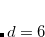(5.54)

where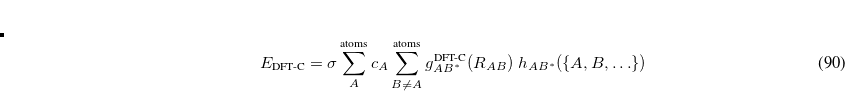is a damped, pairwise BSSE correction,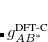(5.55)

The quantity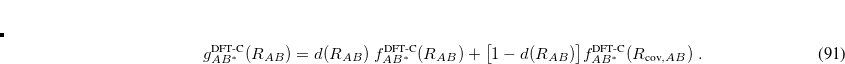(5.56)

is the undamped pairwise BSSE and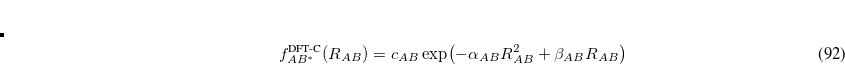(5.57)

is a damping function. The quantity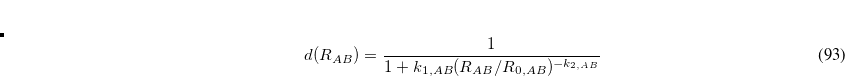is a many-body correction to the two-body BSSE correction, given by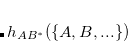(5.58)

where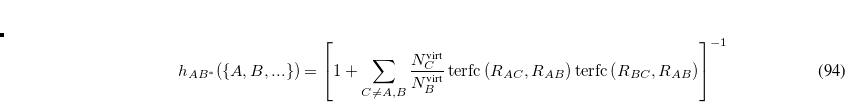(5.59)

The parameters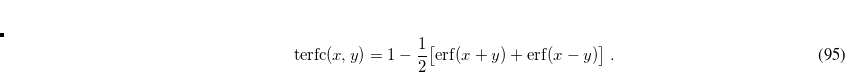,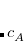,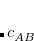, and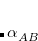are basis-set-dependent, and the overall scaling parameter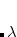is loosely method-dependent. All of these parameters are set internally based on the method and basis $rem specifications. Note: Currently, only the def2-SVPD basis set is supported for use with DFT-C. The DFT-C correction is governed by the$rem variable DFT_C; to invoke the DFT-C method, simply add this to your input:

DFT_C    TRUE


The DFT-C method can be applied to any local, GGA, or meta-GGA density functional, as in the following example.

Example 5.54  Geometry optimization of the methane dimer using B97M-V-C/def2-SVPD, i.e., the B97M-V functional with the DFT-C BSSE correction in the def2-SVPD basis set.

$molecule 0 1 C 0.000000 -0.000140 1.859161 H -0.888551 0.513060 1.494685 H 0.888551 0.513060 1.494685 H 0.000000 -1.026339 1.494868 H 0.000000 0.000089 2.948284 C 0.000000 0.000140 -1.859161 H 0.000000 -0.000089 -2.948284 H -0.888551 -0.513060 -1.494685 H 0.888551 -0.513060 -1.494685 H 0.000000 1.026339 -1.494868$end

$rem JOBTYPE opt BASIS def2-SVPD METHOD b97m-v DFT_C true$end


DFT_C
 Controls whether the DFT-C empirical BSSE correction should be added.

TYPE:
 LOGICAL

DEFAULT:
 FALSE

OPTIONS:
 FALSE (or 0) Do not apply the DFT-C correction TRUE (or 1) Apply the DFT-C correction

RECOMMENDATION:
 NONE